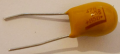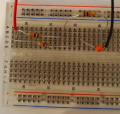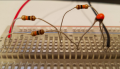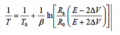# NTC Thermistor Voltage-Temperature Measurement

#### Claudia Cardona

Joined Feb 25, 2018
1
I'm working on a NTC Thermistor circuit attached to a 9 Volt battery, I'm trying to read the voltage and from there convert that reading into temperature. I made a Wheatstone Bridge Circuit to help me do that. My goal is to be able to measure the voltage change with minimal fluctuations when measuring with the multimeter and have an accurate measurement.A couple questions:
To prevent the thermistor from self heating should I add a capacitor? If so where could I place the capacitor on the breadboard?

I am using 3 (10kohm) resistors, is that a good quantity for the circuit built?

The thermistor used is NTC thermistor (NTCLE100E3) of R25= 10000 ohms and a B25/85= 3977K

Theres a formula I saw on Ametherm to calculate the Temperature, what would Rb and R0 represent? Also change in voltage for both top and bottom would the same or different? The temperature measured will be between 0ºC- 25ºC.#### MrChips

Joined Oct 2, 2009
19,416
You don't need a Wheatstone bridge.
Simply measure the voltage across the thermistor.
Use Ohm's law to calculate the resistance of the thermistor.
Go to the data sheet of the thermistor and enter the characteristic curve of your thermistor into a spreadsheet. Perform a linear least squares fit to the data and obtain an expression for temperature as a function of resistance.